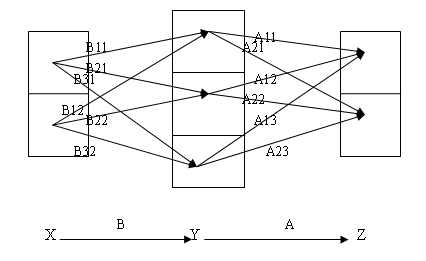# 图示矩阵分块乘法# 点集拓扑要义（二）

（五）拓扑空间中的序列极限与集合聚点

# 隐函数定理的补充，隐函数可导性与可微性的讨论

i) $$F(x_0,y_0)=0$$
ii) $$F(x,y)$$ 与 $$F_y(x,y)$$ 在 $$(x_0,y_0)$$ 的某个邻域内连续
iii) $$F_y(x_0,y_0)\not=0$$

1) $$y_0=y(x_0)$$，$$F(x,y(x))=0,\forall xin(x_0-\delta,x_0+\delta)$$
2) $$y(x)$$ 在 $$(x_0-\delta,x_0+\delta)$$ 内连续

iv) $$F_x(x,y)$$ 也在 $$(x_0,y_0)$$ 的一个邻域内连续，则上述的 $$y=y(x)$$ 在 $$x_0$$ 的一个邻域内一阶导数连续，且
$y'(x)=-\frac{F_x(x,y(x))}{F_y(x,y(x))}$

# 偏导数与可微性的进一步讨论

$$F(x_0+h,y_0+k)-F(x_0,y_0)=Ah+Bk+o(\sqrt{h^2+k^2})$$

$$F(x_0+h,y_0+k)-F(x_0,y_0)=Ah+Bk+\alpha h+\beta k$$

$\lim_{(h,k)\to(0,0)}\alpha(h,k)=\lim_{(h,k)\to(0,0)}\beta(h,k)=0$